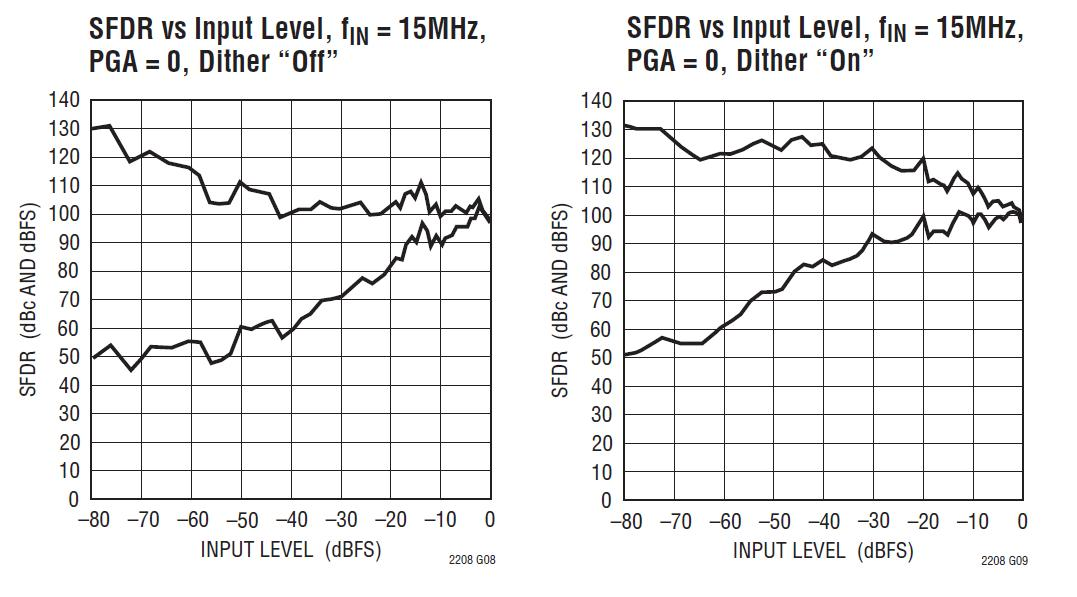# Internal Dither Improves High Speed ADC SFDR Performance

Click here for a complete list of analog to digital converters

The LTC2208 is a 16-bit ADC with a very linear transfer function; however, at low input levels even slight imperfections in the transfer function will result in unwanted tones. Small errors in the transfer function are usually a result of ADC element mismatches. An optional internal dither mode can be enabled to randomize the input location on the ADC transfer curve, resulting in improved SFDR for low signal levels. As shown in the following figure, the output of the sample-and-hold amplifier is summed with the output of a dither DAC.Functionally Equivalent Diagram of Internal Dither Circuitry

The dither DAC is driven by a long sequence pseudo-random number generator; the random number fed to the dither DAC is also subtracted from the ADC result. If the dither DAC is precisely calibrated to the ADC, very little of the dither signal will be seen at the output. The dither signal that does leak through will appear as white noise. The dither DAC is calibrated to result in less than 0.5dB elevation in the noise floor of the ADC, as compared to the noise floor with dither off.LTC2208 FFT Plot With –20dBFS Input Signal: Internal Dither Turned On Versus Internal Dither Turned Off

Note in these two graphs, the input signal is small (–20dBFS) as this is where the effect of the dither is most visible. The spurs seen with the dither "On" are greatly reduced.

In the LTC2208 datasheet, you can also see how the dither improvement varies with the analog input signal level. The figure below shows the SFDR with the dither off and with dither on (Note the Y axis gives both SFDR in dBFS and dBc. dBc is the higher of the two graph lines: simply add the analog input level from the x-axis (dBFs) to the SFDR(dBFs) to obtain the dBc value.). Though both plots start and end at the same value, the plot with the dither "on" has much higher values for the SFDR between the end points.LTC2208 SFDR vs. Input Signal Level Plot: Internal Dither Turned On Versus Internal Dither Turned Off